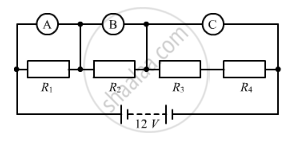Share

# The Resistors R1, R2, R3 And R4 In the Figure Given Below Are All Equal in Value. - CBSE Class 10 - Science

#### Question

The resistors R1, R2, R3 and R4 in the figure given below are all equal in value.What would you expect the voltmeter A, B and C to read assuming that the connecting wires in the circuit have negligible resistance?

#### Solution

The p.d across all the resistors R1R2R3 and R4 is 12 V.
Since all the resistors are of the same value, the p.d at exactly half of the combination, i.e. the voltage shown by the voltmeter C will be 6 V. Similarly, the p.d. shown by the voltmeters A and B is one-fourth the p.d. across all the resistors. Therefore, A and B will have a reading of 3 V.

Is there an error in this question or solution?

#### Video TutorialsVIEW ALL 

Solution The Resistors R1, R2, R3 And R4 In the Figure Given Below Are All Equal in Value. Concept: Ohm’s Law.
S